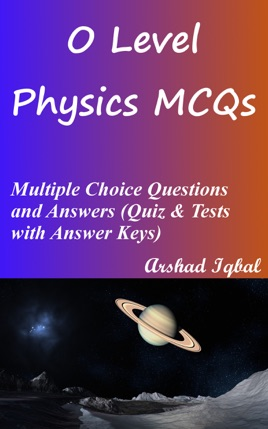• 4,49 €

## Descrizione dell’editore

O Level Physics Multiple Choice Questions and Answers pdf: MCQs, Quizzes & Practice Tests. O level physics quiz questions and answers pdf with practice tests for online exam prep and job interview prep. O level physics study guide with questions and answers about electromagnetic waves, energy, work and power, forces, general wave properties, heat capacity, kinematics, kinetic theory of particles, light, mass, weight and density, measurement of physical quantities, measurement of temperature, measurements, melting and boiling, pressure, properties and mechanics of matter, simple kinetic theory of matter, sound, speed, velocity and acceleration, temperature, thermal energy, thermal properties of matter, transfer of thermal energy, turning effects of forces, waves physics.
O level physics questions and answers to get prepare for career placement tests and job interview prep with answers key. Practice exam questions and answers about physics, composed from O level physics textbooks on chapters:
Electromagnetic Waves Multiple Choice Questions: 17 MCQs
Energy, Work and Power Multiple Choice Questions: 89 MCQs
Forces Multiple Choice Questions: 80 MCQs
General Wave Properties Multiple Choice Questions: 16 MCQs
Heat Capacity Multiple Choice Questions: 11 MCQs
Kinematics Multiple Choice Questions: 30 MCQs
Kinetic Theory of Particles Multiple Choice Questions: 47 MCQs
Light Multiple Choice Questions: 45 MCQs
Mass, Weight and Density Multiple Choice Questions: 39 MCQs
Measurement of Physical Quantities Multiple Choice Questions: 6 MCQs
Measurement of Temperature Multiple Choice Questions: 18 MCQs
Measurements Multiple Choice Questions: 26 MCQs
Melting and Boiling Multiple Choice Questions: 23 MCQs
Pressure Multiple Choice Questions: 47 MCQs
Properties and Mechanics of Matter Multiple Choice Questions: 7 MCQs
Simple Kinetic Theory of Matter Multiple Choice Questions: 16 MCQs
Sound Multiple Choice Questions: 16 MCQs
Speed, Velocity and Acceleration Multiple Choice Questions: 7 MCQs
Temperature Multiple Choice Questions: 99 MCQs
Thermal Energy Multiple Choice Questions: 48 MCQs
Thermal Properties of Matter Multiple Choice Questions: 140 MCQs
Transfer of Thermal Energy Multiple Choice Questions: 10 MCQs
Turning Effects of Forces Multiple Choice Questions: 37 MCQs
Waves Physics Multiple Choice Questions: 22 MCQs
O level physics interview questions and answers on acceleration free fall, acceleration of freefall, acceleration: o level physics, atmospheric pressure and weather, balanced forces and unbalanced forces, boiling and condensation, boiling point, center of gravity and stability, center of gravity.
O level physics test questions and answers on condensation, conduction, conduction, convection, converging lens, density: o level physics, displacement-time graph, distance, time and speed, effects of forces on motion, efficiency, electromagnetic waves, energy and units, energy, work and power, energy: o level physics.
O level physics exam questions and answers on evaporation, evidence of molecular motion, force, forces and effects, forces and motion, gravity, heat capacity: physics, heat capacity: water and air, hydraulic systems, inertia, mass and weight, introducing waves, introduction to forces, introduction to light, introduction to pressure, introduction to sound, introduction to waves, kinetic molecular model of matter.
O level physics objective questions and answers on kinetic theory, latent heat, mass and weight, mass, weight and density, measurement of density, measuring atmospheric pressure, measuring temperature, measuring time, melting and solidification, meter rule and measuring tape, moments, o level online exam test, physical quantities and SI unit, physics of light, physics of temperature, power, precision and range, pressure in gases, pressure in liquids, pressure of gases, pressure physics, principle of moment, properties of wave motion, radiation, rate of infrared radiations.